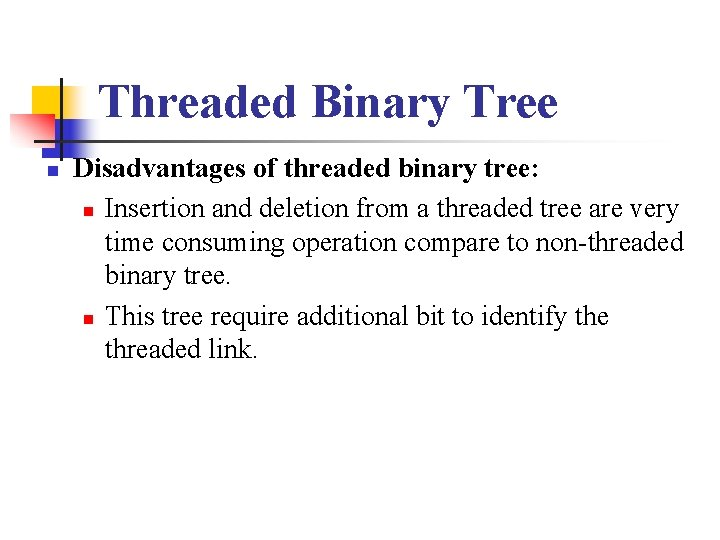• Slides: 19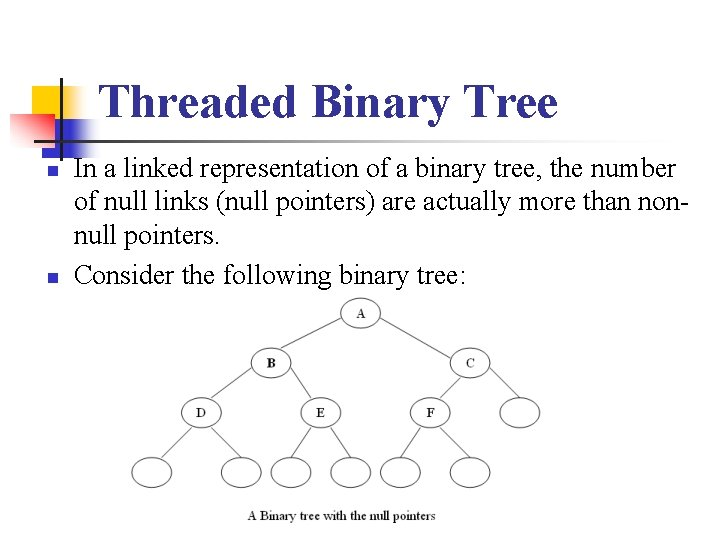Threaded Binary Tree n n In a linked representation of a binary tree, the number of null links (null pointers) are actually more than nonnull pointers. Consider the following binary tree: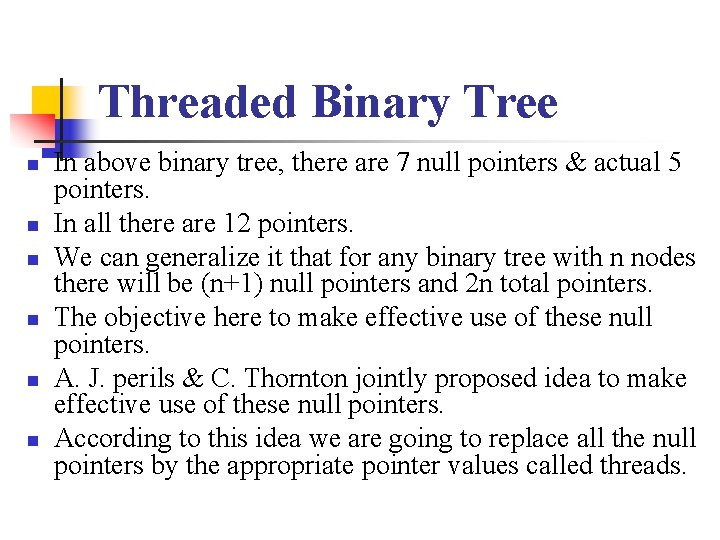Threaded Binary Tree n n n In above binary tree, there are 7 null pointers & actual 5 pointers. In all there are 12 pointers. We can generalize it that for any binary tree with n nodes there will be (n+1) null pointers and 2 n total pointers. The objective here to make effective use of these null pointers. A. J. perils & C. Thornton jointly proposed idea to make effective use of these null pointers. According to this idea we are going to replace all the null pointers by the appropriate pointer values called threads.Threaded Binary Tree n n And binary tree with such pointers are called threaded tree. In the memory representation of a threaded binary tree, it is necessary to distinguish between a normal pointer and a thread. Original tree is the left subtree of the header node. Empty binary tree is given below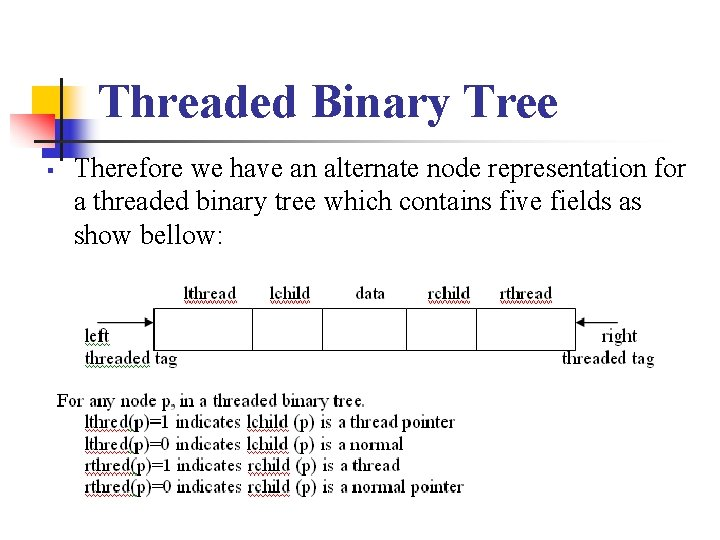Threaded Binary Tree Therefore we have an alternate node representation for a threaded binary tree which contains five fields as show bellow:Threaded Binary Tree n n Also one may choose a one-way threading or a two-way threading. Here, our threading will correspond to the in order traversal of T.Threaded Binary Tree One-Way n n Accordingly, in the one way threading of T, a thread will appear in the right field of a node and will point to the next node in the in-order traversal of T. See the bellow example of one-way in-order threading.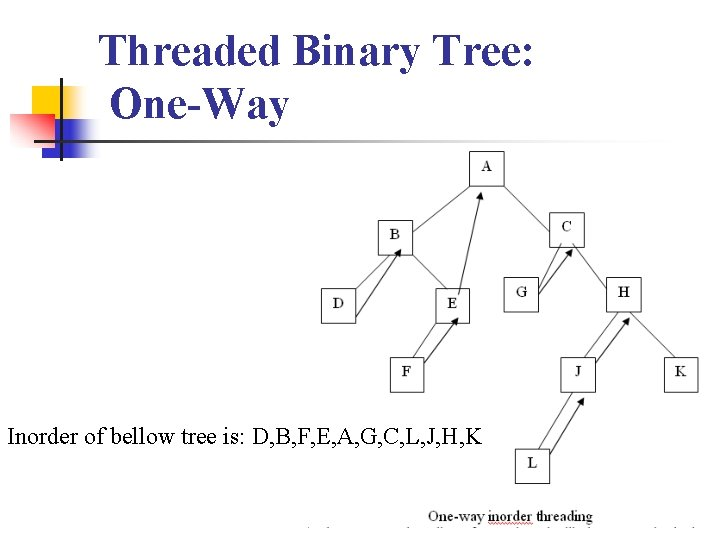Threaded Binary Tree: One-Way Inorder of bellow tree is: D, B, F, E, A, G, C, L, J, H, KThreaded Binary Tree Two-Way n n n In the two-way threading of T. A thread will also appear in the left field of a node and will point to the preceding node in the in-order traversal of tree T. Furthermore, the left pointer of the first node and the right pointer of the last node (in the in-order traversal of T) will contain the null value when T does not have a header node.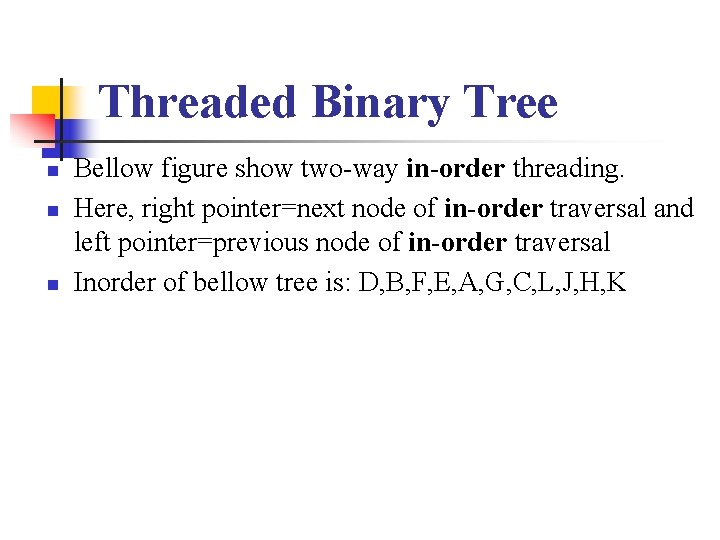Threaded Binary Tree n n n Bellow figure show two-way in-order threading. Here, right pointer=next node of in-order traversal and left pointer=previous node of in-order traversal Inorder of bellow tree is: D, B, F, E, A, G, C, L, J, H, KThreaded Binary Tree Two-way Threading with Header node n Again two-way threading has left pointer of the first node and right pointer of the last node (in the inorder traversal of T) will contain the null value when T will point to the header nodes is called two-way threading with header node threaded binary tree.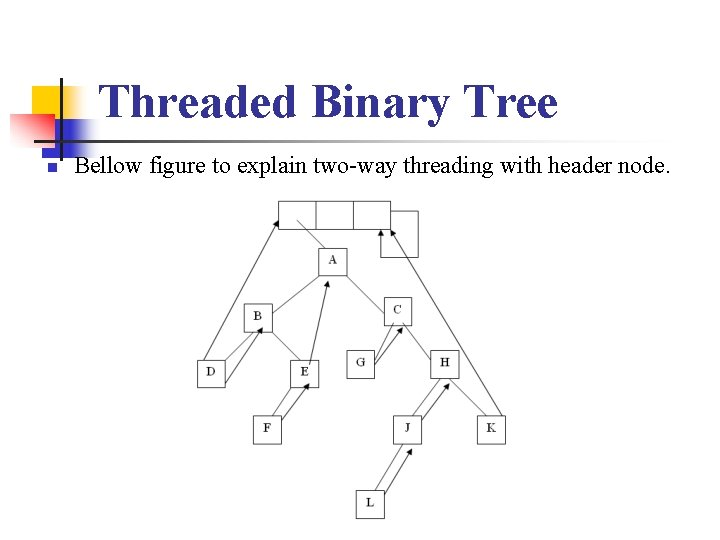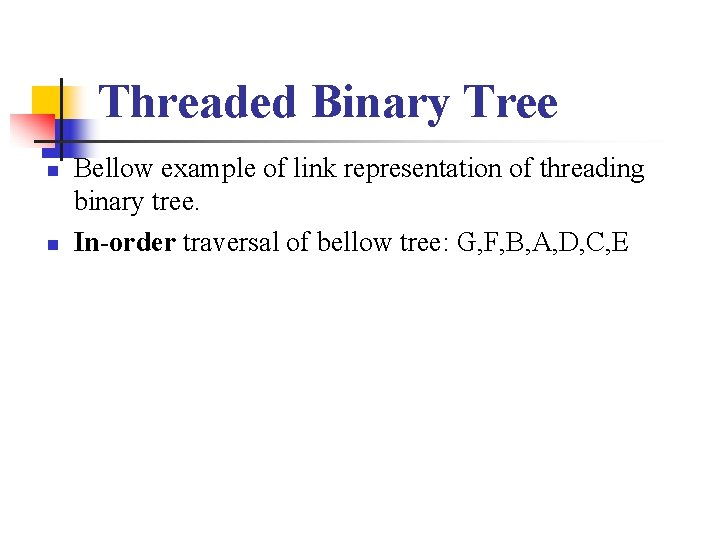Threaded Binary Tree n n Bellow example of link representation of threading binary tree. In-order traversal of bellow tree: G, F, B, A, D, C, E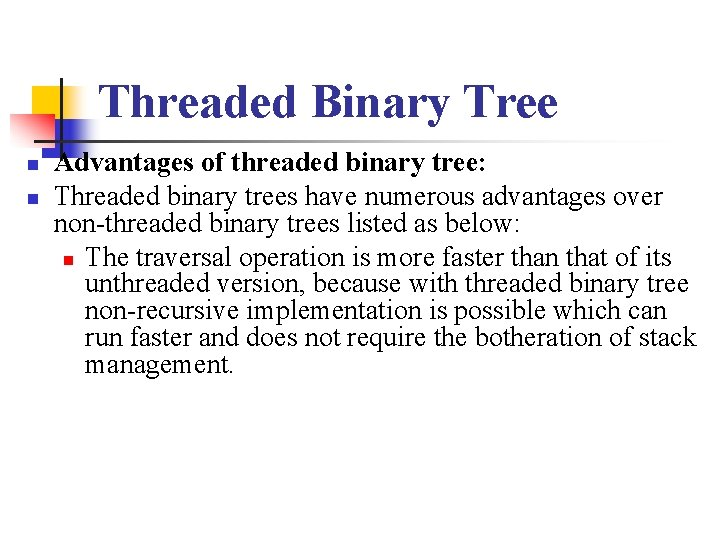Threaded Binary Tree n n Advantages of threaded binary tree: Threaded binary trees have numerous advantages over non-threaded binary trees listed as below: n The traversal operation is more faster than that of its unthreaded version, because with threaded binary tree non-recursive implementation is possible which can run faster and does not require the botheration of stack management.Threaded Binary Tree n Advantages of threaded binary tree: n The second advantage is more understated with a threaded binary tree, we can efficiently determine the predecessor and successor nodes starting from any node. In case of unthreaded binary tree, however, this task is more time consuming and difficult. For this case a stack is required to provide upward pointing information in the tree whereas in a threaded binary tree, without having to include the overhead of using a stack mechanism the same can be carried out with the threads.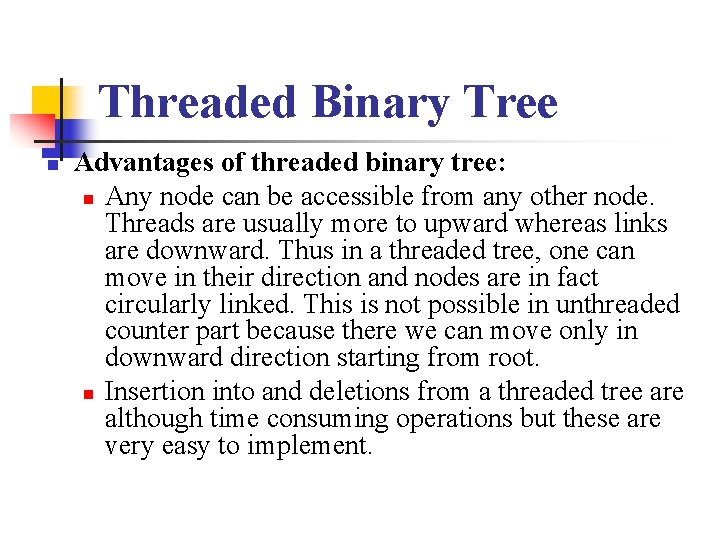Threaded Binary Tree n Advantages of threaded binary tree: n Any node can be accessible from any other node. Threads are usually more to upward whereas links are downward. Thus in a threaded tree, one can move in their direction and nodes are in fact circularly linked. This is not possible in unthreaded counter part because there we can move only in downward direction starting from root. n Insertion into and deletions from a threaded tree are although time consuming operations but these are very easy to implement.